Next: Building a more complex Up: DECONVOLUTION WITH A KNOWN Previous: DECONVOLUTION WITH A KNOWN

## The basic operator

If we consider the simple case in whichis a sufficiently sparse series (so that the number of samples between any two adjacent non-zero-valued samples is at least equal to the number of samples of the wavelet, i.e. Lw), it is possible to devise a simple operator to retrieve:(2)

The symbol stands for correlation, while the operatoris defined as follows: The result of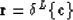is given by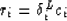whereWhen the seriesis still sparse but the temporal distance between non-vanishing-samples is larger than the minimum distance still resolvable by the wavelet (that is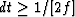, where f is the highest frequency component of the wavelet), the operator in equation (2) will not give the exact solution. Here however, an exact solution can still be obtained through an iterative process. Let's consider equation (2) as the zero-order approximation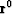to the time series: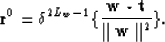(3)

The residual between the original trace and the trace predicted by this zero-order approximation for the reflectivity will be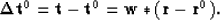(4)

Because the operator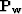in equation (2) is an approximation to the inverse of the convolution by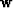, we can apply it to equation (4) to get a zero-order approximation to: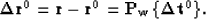(5)

Therefore, the first-order approximation for the reflectivity series will be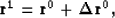and in general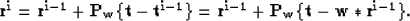(6)

In Figure 1 this iterative process is applied to a synthetic trace whose original reflectivity series is comprised of sparse spikes. The distance between some of the spikes is successively decreased to show how the relaxation of the sparseness condition affects the convergence of the method. Except for (c), the exact solution was reached in a few number of iterations, even when the separation between adjacent spikes is reduced to one third of the wavelet size. Because in (c) the exact solution was not reached within a finite number of iterations (though an extremely close solution was found after a few iterations), it will be necessary to improve the basic operator Pw if we intend to apply the method to more complex time series.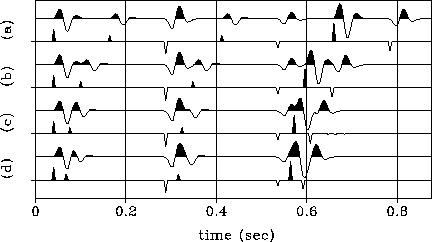sparse
Figure 1
The iterative process described by equation (6) is applied to four synthetic traces. The traces differ only in the distance between some of the spikes of the associated reflectivity series. (a) When the distance between spikes is around 1.5 times the wavelet's size the exact reflectivities are retrieved on the 0-order iteration (that is, equation 2). As the distance between some of the spikes decreases, the number of iterations necessary to get the exact solution increases: 1 iteration in (b) and 8 iterations in (d). Although (c) has a fast convergence (the sixth iteration is shown), the exact solution is not reached, as we can see by the small residuals beyond 0.6 seconds.Next: Building a more complex Up: DECONVOLUTION WITH A KNOWN Previous: DECONVOLUTION WITH A KNOWN
Stanford Exploration Project
1/13/1998